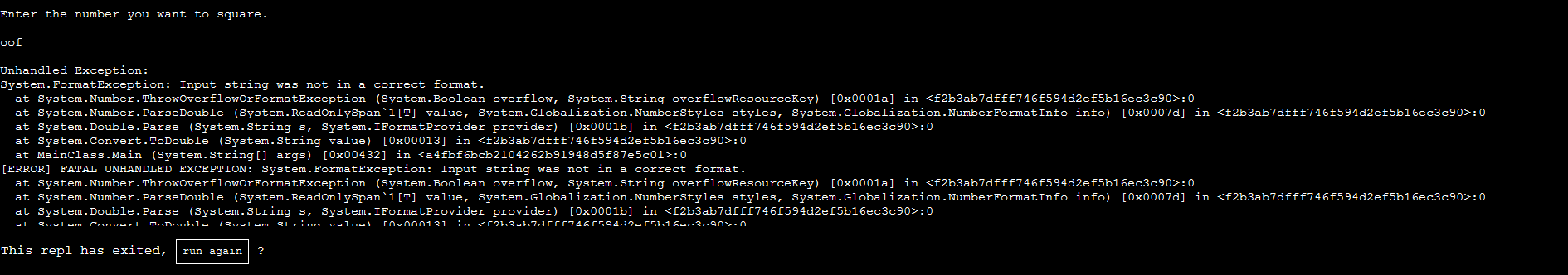Share your repls and programming experiences

← Back to all posts
##### CALCULATOR# - C# calculator in console

`CALCULATOR#` is a C# calculator in the console where you can do the following.

• Add 2 numbers
• Subtract a number from a number
• Multiply 2 numbers
• Divide a number by a number
• Division with decimal points
• Division with remainders
• Get the square root of a number
• Get the cube root of a number
• Square a number
• Cube a number
• Compare two numbers
• Get the absolute value of a number
• Raise a number to the power you choose

This program was made with the help of the Microsoft Online C# .NET Documentation, like most other C# programs.

### AND BE SURE TO ONLY USE NUMBERS AND DECIMAL POINTS! If you use any non-number or non-decimal-point characters, the program will throw an exception.

An example of that run's terrible fate:You may want to zoom in.

This may be more boring than my other programs, but hope you find it useful anyway.